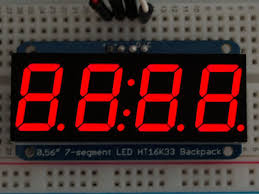# Jarvis and Seven Segments

## problem:-

All over the world, peoples are working on energy solution. It would be a tough time for our next generation to survive if we don’t think about solution. Tony stark is working on a new project and wants to display his project using “seven segment display – concept”. Tony Stark gave Jarvis a task to find a number from his Favorite list of number for which the energy consumption is lowest.
(Assuming that for a digit to represent Tony stark is using 7 bulbs and only those bulbs light up which are required to represent a number and rest other would be completely off.)
Help Jarvis and conserve energy.
Seven segment display – https://en.wikipedia.org/wiki/Seven-segment_displayInput:
First line will contain the number of test cases and for every test case first line will contain length of favorite list and the second line for a test case will contain n numbers
Output:
For every test case print the answer. If there exist more than 1 numbers for which same number of bulbs are required than output the number which occurs first in the Favorite list.
Constraints:
Test cases< 10
A[i] < 10^6
Size of list < 10^5
SAMPLE INPUT

`151 2 3 4 5`
SAMPLE OUTPUT

`1`
Explanation

## Number 1 needs only two bulbs to represent.Solution:-

#include<stdio.h>
int main()
{
long int t,n,r,sum=0,ans,flag=0,i,j;
long int a,new_num,n1;
scanf(“%ld”,&t);
for(i=0;i<t;i++)
{
flag=0;
scanf(“%ld”,&n);
for( j=0;j<n;j++)
{
scanf(“%ld”,&a);
n1=a;
if(a==0)
sum=sum+6;
while(a!=0)
{
r=a%10;
if(r==1)
sum=sum+2;
if(r==2||r==3||r==5)
sum=sum+5;
if(r==4)
sum=sum+4;
if(r==6||r==9||r==0)
sum=sum+6;
if(r==7)
sum=sum+3;
if(r==8)
sum=sum+7;
a=a/10;
}

if(flag==0)
{
ans=sum;
new_num=n1;
flag=1;
}
if(ans>sum)
{
ans=sum;
new_num=n1;
}
sum=0;
}
printf(“%ldn”,new_num);
}
return 0;
}